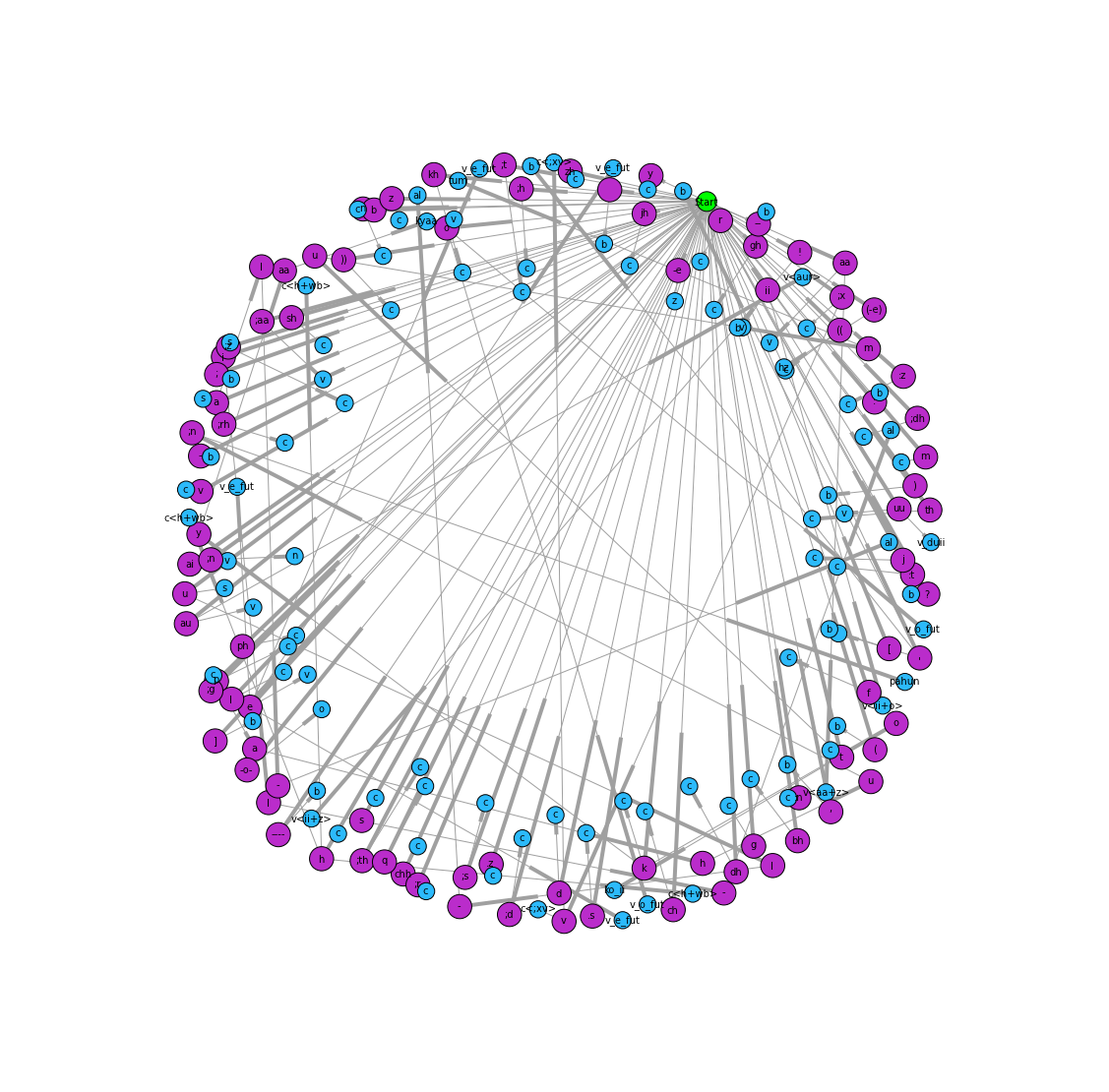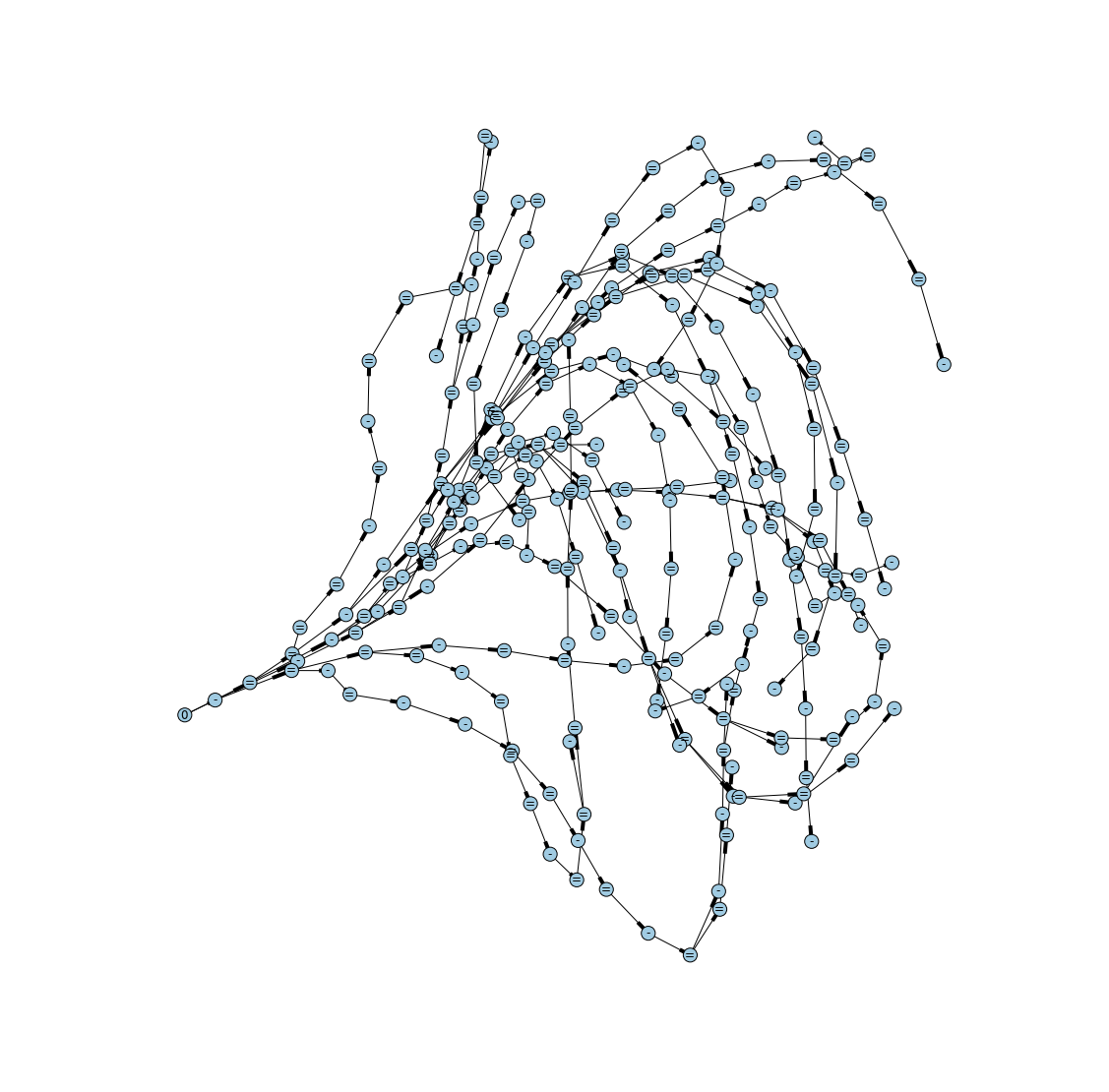## "Bioinformatic Approaches to the Computation of Poetic Meter"¶

IPython Notebook for presentation at Shared Horizons: Data, Biomedicine and the Digital Humanities
@ University of Maryland, 12 April 2013
A. Sean Pue, Tracy K. Teal, C. Titus Brown
Michigan State University

In :
# import some modules

import settings
from meter_graph import MeterGraph
import graph_parser
from graph_parser import GraphParser
from pprint import pprint
import meter_graph


## Transcription¶

Convert from transliteration to metrical components

ranj se ;xuugar hu))aa insaa;n to mi;t jaataa hai ranj => bcsccbcvbcvcscbcscvbsccvnbcvbcscbcvcvbcvbcscc

b = word break, c = consonant, v = long vowel, s = short vowel, etc.

In :
# loads rules for transcribing text into components of meter
# e.g. b = break, c = consonant, v = long vowel, s = short vowel
# rules can include previous tokens, class of token before previous, following tokens, class of token following tokens
pp = GraphParser('settings/urdu-meter.yaml')
pprint(pp.rules[-5:])

def transcribe(s):
return pp.parse(s)

(ParserRule(production='pahun', prev_class='wb', prev_tokens=None, tokens=['p', 'a', 'h', 'u', ';n'], next_tokens=['ch'], next_class='vowel'),
ParserRule(production='kyaa', prev_class='wb', prev_tokens=None, tokens=['k', 'y', 'aa'], next_tokens=None, next_class='wb'),
ParserRule(production='al', prev_class='wb', prev_tokens=None, tokens=['u', 'l', '-'], next_tokens=None, next_class=None),
ParserRule(production='al', prev_class='wb', prev_tokens=None, tokens=['i', 'l', '-'], next_tokens=None, next_class=None),
ParserRule(production='tum', prev_class='wb', prev_tokens=None, tokens=['t', 'u', 'm'], next_tokens=['h'], next_class=None))

In :
transcription = transcribe(' ranj se ;xuugar hu))aa insaa;n to mi;t jaataa hai ranj')
transcription.output # output of transcript

Out:
'bcsccbcvbcvcscbcscvbsccvnbcvbcscbcvcvbcvbcscc'
In :
# also stores details of matches for later
transcription.matches[0:5]

Out:
[ParserRule(production='b', prev_class=None, prev_tokens=None, tokens=' ', next_tokens=None, next_class=None),
ParserRule(production='c', prev_class=None, prev_tokens=None, tokens=['r'], next_tokens=None, next_class=None),
ParserRule(production='s', prev_class=None, prev_tokens=None, tokens=['a'], next_tokens=None, next_class=None),
ParserRule(production='c', prev_class=None, prev_tokens=None, tokens=['n'], next_tokens=None, next_class=None),
ParserRule(production='c', prev_class=None, prev_tokens=None, tokens=['j'], next_tokens=None, next_class=None)]
In :
# Using a graph here
# Constraints on final edge (previous token class, previous tokens, next tokens, next token class)
graph_parser.draw_parser_graph(pp.DG)## Parsing and Translation¶

Parses and translates into known meters,
e.g bcsccbcvbcvcscbcscvbsccvnbcvbcscbcvcvbcvbcscc => =-==/=-==/=-==/=-=- = is long, - is short, / is foot-divider

In :
mg = MeterGraph()

def translate(s):
return mg.graph_scan(s)

In :
example=' ranj se ;xuugar hu))aa insaa;n to mi;t jaataa hai ranj'
translations = translate(example)
for t in translations:
print "scan: ",t.scan, "meter id: ",t.meter_type
print "Also saves matches, e.g.:"
pprint(t.matches[0:5], indent=4)


scan:  =-===-===-===-=- meter id:  G1
Also saves matches, e.g.:
[   NodeMatch(node_type='=', matched_tokens=['b', 'c', 's', 'c'], node_id=0, orig_tokens=[' ', ['r'], ['a'], ['n']], found='l_bcsc', token_i=0),
NodeMatch(node_type='-', matched_tokens=['c'], node_id=1, orig_tokens=[['j']], found='s_c', token_i=4),
NodeMatch(node_type='=', matched_tokens=['b', 'c', 'v'], node_id=11, orig_tokens=[' ', ['s'], ['e']], found='l_bcv', token_i=5),
NodeMatch(node_type='=', matched_tokens=['b', 'c', 'v'], node_id=43, orig_tokens=[' ', [';x'], ['uu']], found='l_bcv', token_i=8),
NodeMatch(node_type='=', matched_tokens=['c', 's', 'c'], node_id=44, orig_tokens=[['g'], ['a'], ['r']], found='l_csc', token_i=11)]

In :
# Using a graph here
# On the edges between nodes are bad matches, e.g. illegal combos
mg.draw_graph()In :
import pydot
import networkx
g=mg.DG
labels=dict((n,d['type']) for n,d in g.nodes(data=True))
networkx.drawing.nx_pylab.draw_graphviz(g, labels=labels, node_size=200)